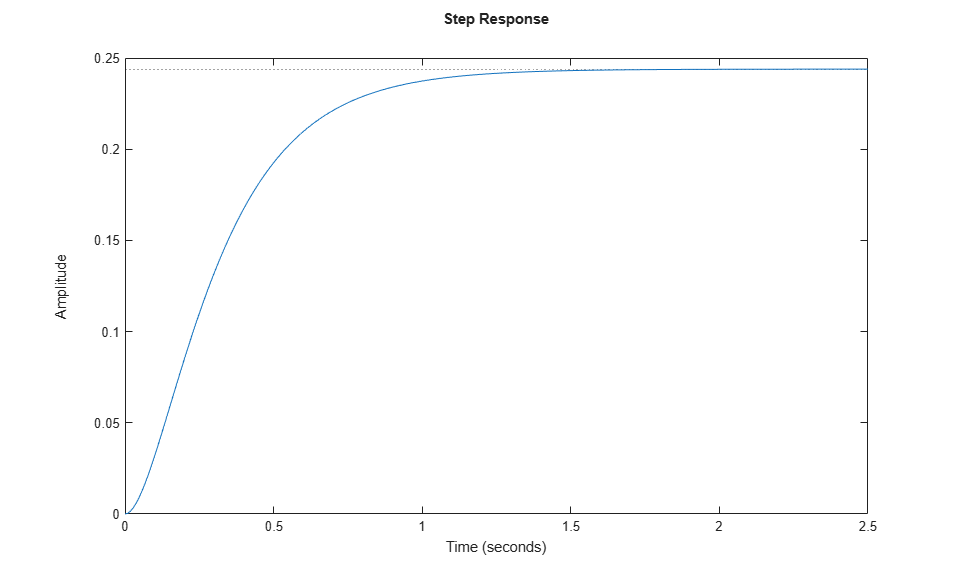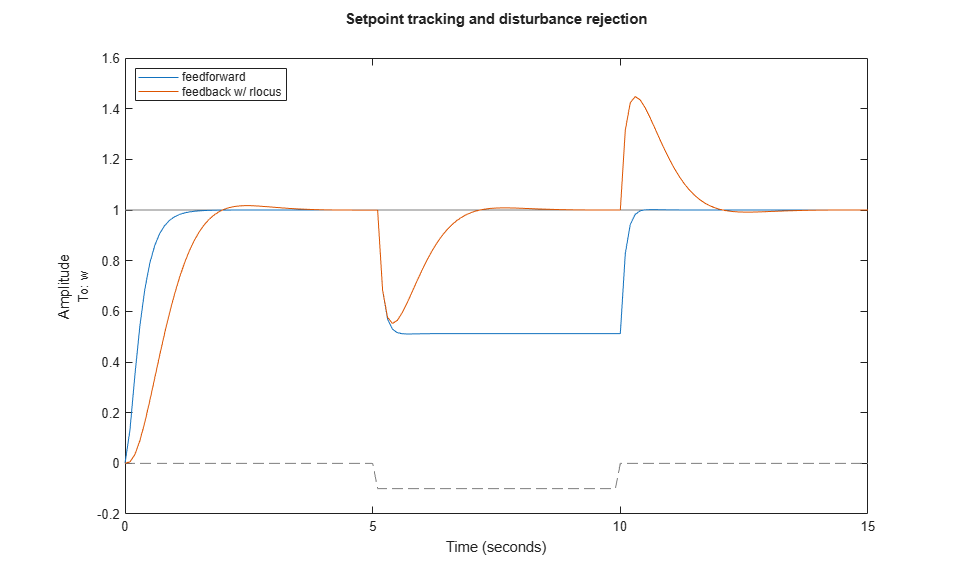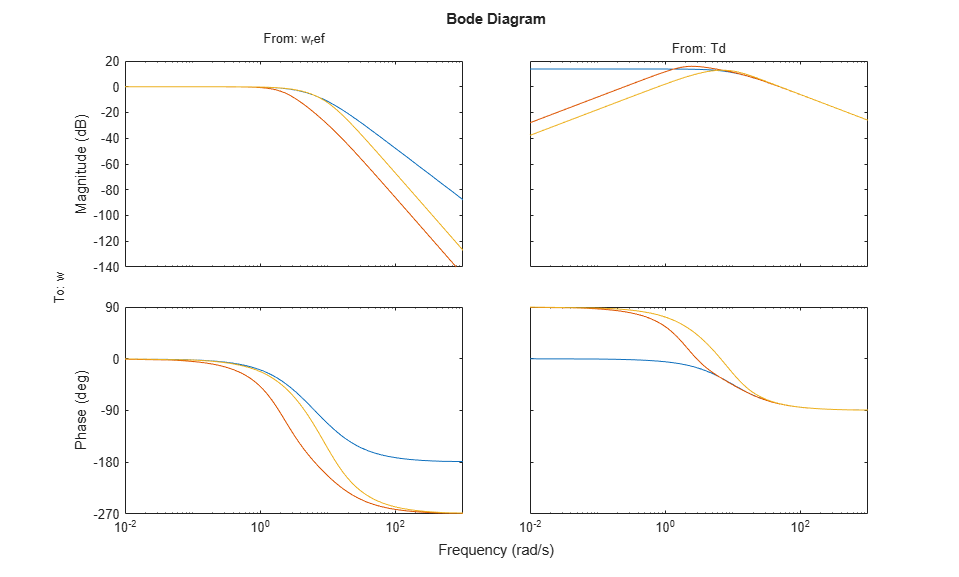# DC Motor Control

This example shows the comparison of three DC motor control techniques for tracking setpoint commands and reducing sensitivity to load disturbances:

• feedforward command

• integral feedback control

• LQR regulation

See "Getting Started:Building Models" for more details about the DC motor model.

### Problem Statement

In armature-controlled DC motors, the applied voltage Va controls the angular velocity w of the shaft.This example shows two DC motor control techniques for reducing the sensitivity of w to load variations (changes in the torque opposed by the motor load).A simplified model of the DC motor is shown above. The torque Td models load disturbances. You must minimize the speed variations induced by such disturbances.

For this example, the physical constants are:

R = 2.0; % Ohms L = 0.5; % Henrys Km = 0.1; % torque constant Kb = 0.1; % back emf constant Kf = 0.2; % Nms J = 0.02; % kg.m^2/s^2 

First construct a state-space model of the DC motor with two inputs (Va,Td) and one output (w):

h1 = tf(Km,[L R]); % armature h2 = tf(1,[J Kf]); % eqn of motion dcm = ss(h2) * [h1 , 1]; % w = h2 * (h1*Va + Td) dcm = feedback(dcm,Kb,1,1); % close back emf loop 

Note: Compute with the state-space form to minimize the model order.

Now plot the angular velocity response to a step change in voltage Va:

stepplot(dcm(1));Right-click on the plot and select "Characteristics:Settling Time" to display the settling time.

### Feedforward DC Motor Control Design

You can use this simple feedforward control structure to command the angular velocity w to a given value w_ref.The feedforward gain Kff should be set to the reciprocal of the DC gain from Va to w.

Kff = 1/dcgain(dcm(1)) 
Kff = 4.1000 

To evaluate the feedforward design in the face of load disturbances, simulate the response to a step command w_ref=1 with a disturbance Td = -0.1Nm between t=5 and t=10 seconds:

t = 0:0.1:15; Td = -0.1 * (t>5 & t<10); % load disturbance u = [ones(size(t)) ; Td]; % w_ref=1 and Td cl_ff = dcm * diag([Kff,1]); % add feedforward gain cl_ff.InputName = {'w_ref','Td'}; cl_ff.OutputName = 'w'; h = lsimplot(cl_ff,u,t); title('Setpoint tracking and disturbance rejection') legend('cl\_ff') % Annotate plot line([5,5],[.2,.3]); line([10,10],[.2,.3]); text(7.5,.25,{'disturbance','T_d = -0.1Nm'},... 'vertic','middle','horiz','center','color','r');Clearly feedforward control handles load disturbances poorly.

### Feedback DC Motor Control Design

Next try the feedback control structure shown below.To enforce zero steady-state error, use integral control of the form

 C(s) = K/s
 where K is to be determined.

To determine the gain K, you can use the root locus technique applied to the open-loop 1/s * transfer(Va->w):

h = rlocusplot(tf(1,[1 0]) * dcm(1)); setoptions(h,'FreqUnits','rad/s'); xlim([-15 5]); ylim([-15 15]);Click on the curves to read the gain values and related info. A reasonable choice here is K = 5. Note that the SISO Design Tool offers an integrated GUI to perform such designs (help sisotool for details).

Compare this new design with the initial feedforward design on the same test case:

K = 5; C = tf(K,[1 0]); % compensator K/s cl_rloc = feedback(dcm * append(C,1),1,1,1); h = lsimplot(cl_ff,cl_rloc,u,t); cl_rloc.InputName = {'w_ref','Td'}; cl_rloc.OutputName = 'w'; title('Setpoint tracking and disturbance rejection') legend('feedforward','feedback w/ rlocus','Location','NorthWest')The root locus design is better at rejecting load disturbances.

### LQR DC Motor Control Design

To further improve performance, try designing a linear quadratic regulator (LQR) for the feedback structure shown below.In addition to the integral of error, the LQR scheme also uses the state vector x=(i,w) to synthesize the driving voltage Va. The resulting voltage is of the form

 Va = K1 * w + K2 * w/s + K3 * i
 where i is the armature current.

For better disturbance rejection, use a cost function that penalizes large integral error, e.g., the cost functionwhereThe optimal LQR gain for this cost function is computed as follows:

dc_aug = [1 ; tf(1,[1 0])] * dcm(1); % add output w/s to DC motor model K_lqr = lqry(dc_aug,[1 0;0 20],0.01); 

Next derive the closed-loop model for simulation purposes:

P = augstate(dcm); % inputs:Va,Td outputs:w,x C = K_lqr * append(tf(1,[1 0]),1,1); % compensator including 1/s OL = P * append(C,1); % open loop CL = feedback(OL,eye(3),1:3,1:3); % close feedback loops cl_lqr = CL(1,[1 4]); % extract transfer (w_ref,Td)->w 

This plot compares the closed-loop Bode diagrams for the three DC motor control designs

bodeplot(cl_ff,cl_rloc,cl_lqr);Click on the curves to identify the systems or inspect the data.

### Comparison of DC Motor Control Designs

Finally we compare the three DC motor control designs on our simulation test case:

h = lsimplot(cl_ff,cl_rloc,cl_lqr,u,t); title('Setpoint tracking and disturbance rejection') legend('feedforward','feedback (rlocus)','feedback (LQR)','Location','NorthWest')Thanks to its additional degrees of freedom, the LQR compensator performs best at rejecting load disturbances (among the three DC motor control designs discussed here).